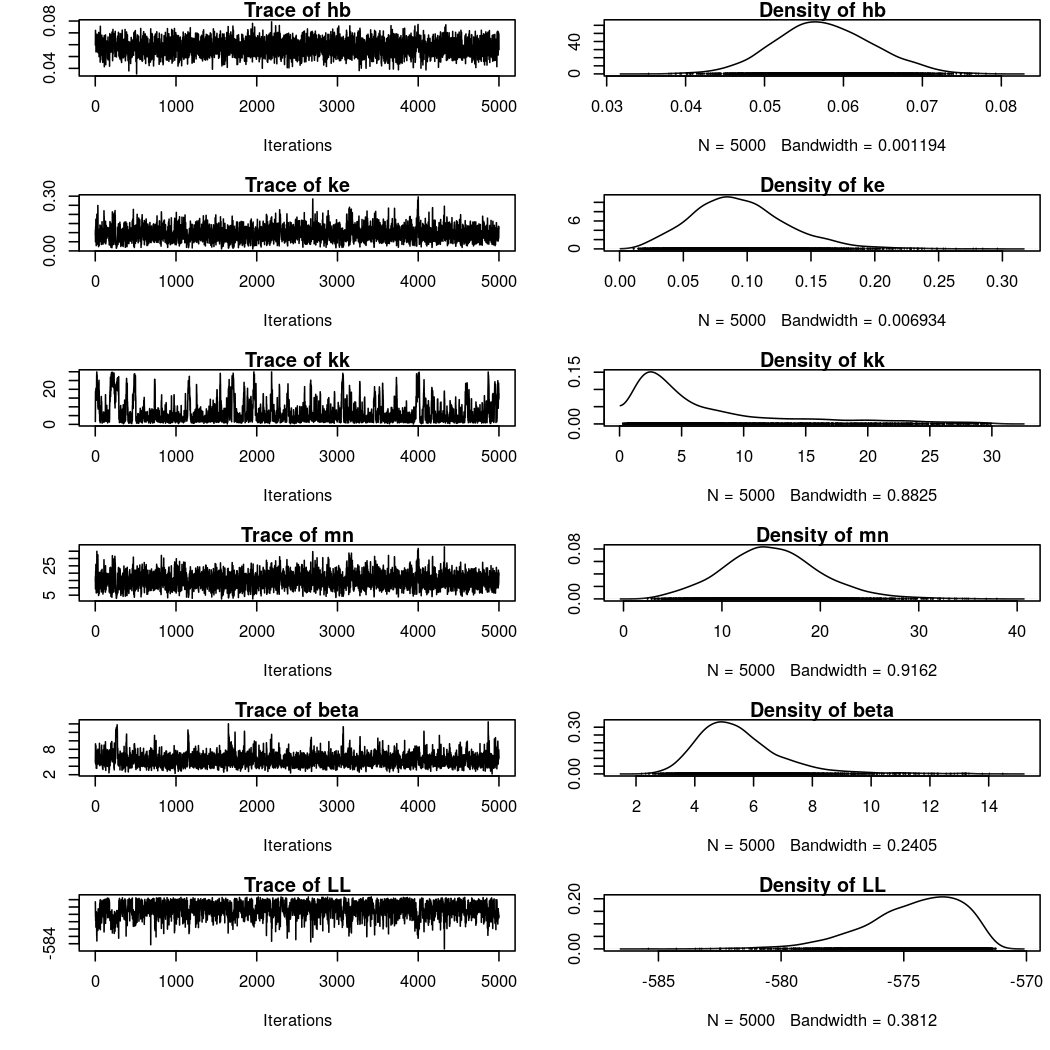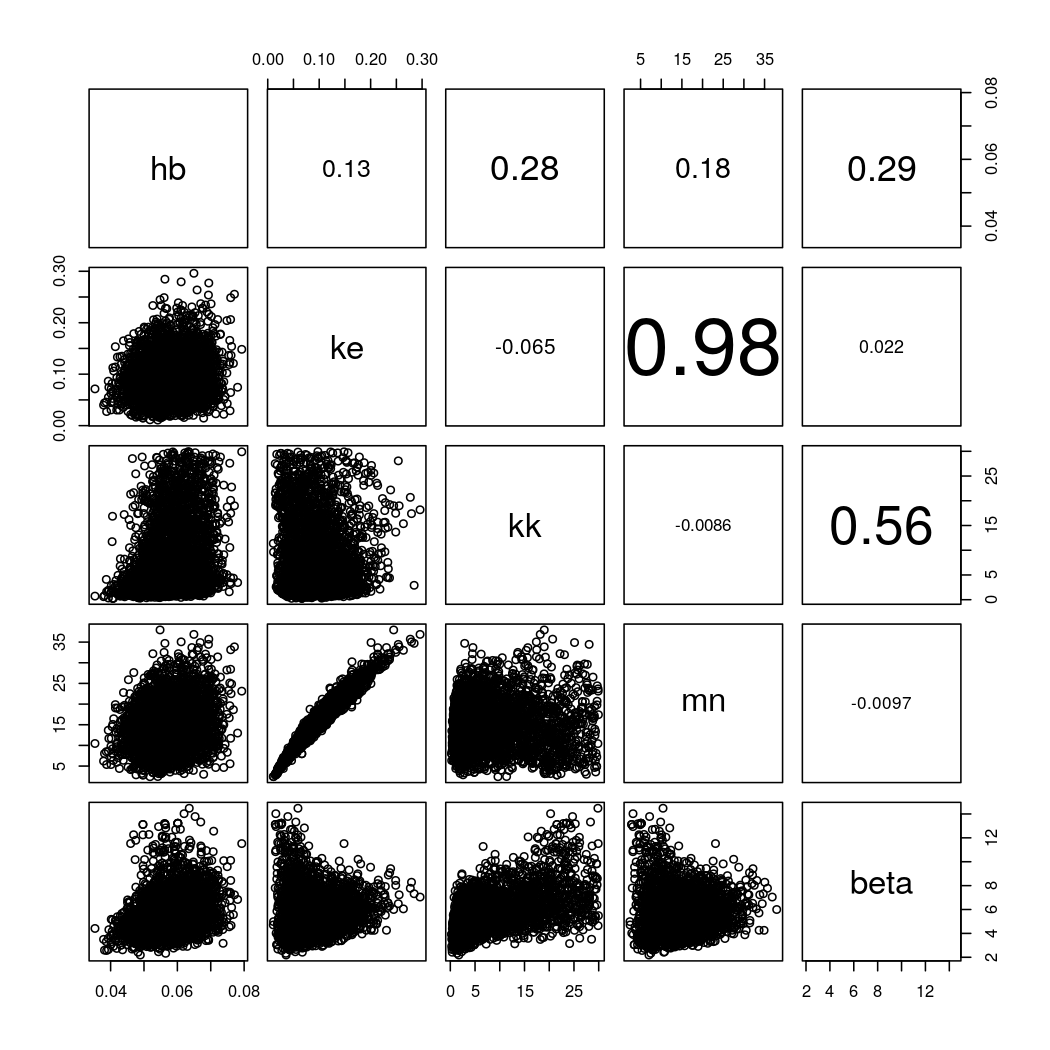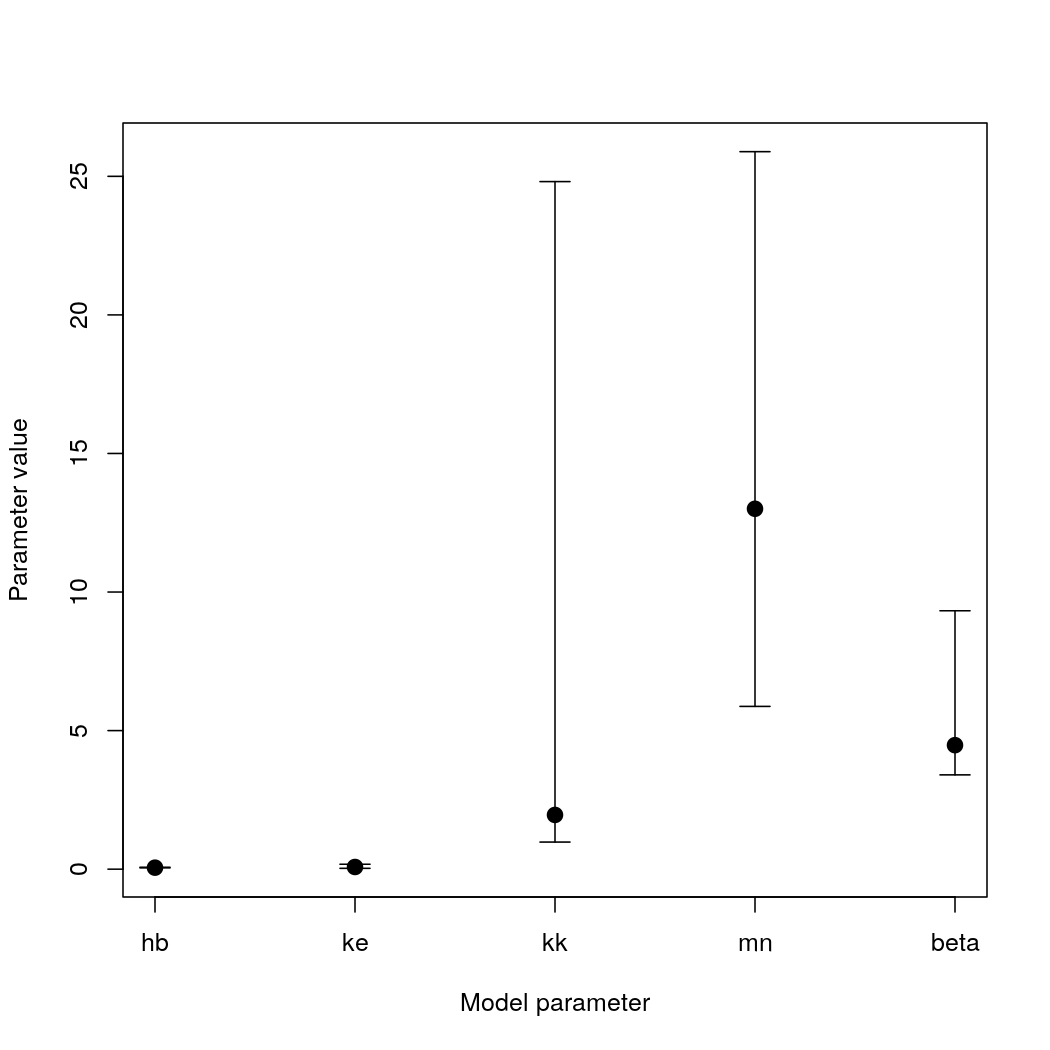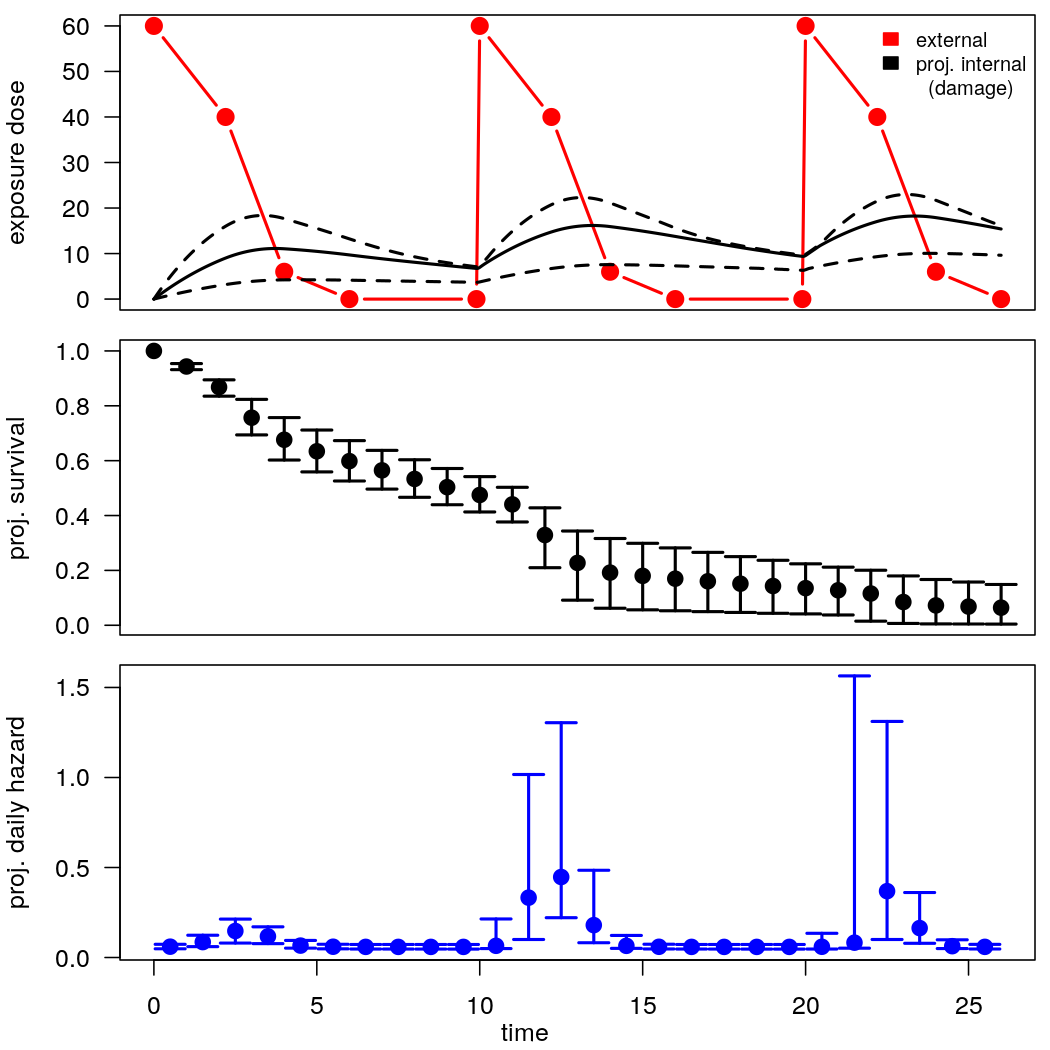# Analysing Diazinon impact on the freshwater crustacean Gammarus pulex using GUTS-SIC-proper

## For performance reasons, this vignette builds from pre-calculated data.
##
##  To run all calculations, set 'do.calc <- TRUE' in the vignette's first code chunk.
##  Building the vignette will take a while.

# Summary

The GUTS-package (Albert et al., 2016) implements the individual tolerance model GUTS-SIC-IT, the stochastic death model GUTS-SIC-SD and the GUTS-SIC-proper model, which combines the GUTS-SIC-IT and the GUTS-SIC-SD model (Jager et al., 2011). We demonstrate a Bayesian model calibration of the proper model on the case study of the freshwater crustacean Gammarus pulex being exposed to Diazinon in three pulsed toxicity tests. This vignette follows the description in Albert et al. (2016), but uses a log-logistic threshold distribution, a slightly different calibration procedure and results analysis. The experiment data is provided with the GUTS-package.

# Prepare

library(GUTS)
packageVersion("GUTS")
#>  '1.1.0'

data(diazinon)
str(diazinon)
#> List of 12
#>  $C1 : num [1:10] 102.7 97.6 0 0 103.9 ... #>$ C2 : num [1:9] 101 106 0 0 104 ...
#>  $C3 : num [1:8] 100.6 94.6 0 0 100.6 ... #>$ Ct1: num [1:10] 0 1.02 1.03 2.99 3.01 ...
#>  $Ct2: num [1:9] 0 1.02 1.03 8 8.01 ... #>$ Ct3: num [1:8] 0 1.02 1.03 16 16.01 ...
#>  $y1 : num [1:23] 70 66 61 55 31 31 29 26 24 22 ... #>$ y2 : num [1:23] 70 65 59 56 54 50 47 46 46 40 ...
#>  $y3 : num [1:23] 70 65 59 55 53 51 48 46 46 46 ... #>$ yt1: int [1:23] 0 1 2 3 4 5 6 7 8 9 ...
#>  $yt2: int [1:23] 0 1 2 3 4 5 6 7 8 9 ... #>$ yt3: int [1:23] 0 1 2 3 4 5 6 7 8 9 ...

# Set up the guts object

Setting up a GUTS-Proper-object requires for each toxicity test

• the exposure profile containing the measured concentration C at each concentration measurement time Ct
• the survival data consisting of the number of survived organisms y at each abundance measurement time yt
• the GUTS-model type model = "Proper"
• the tolerance threshold distribution dist = "loglogistic"

We generate three GUTS-proper-objects; one for each of the toxicity test. The GUTS-objects are collected in a list.

guts_object <- list(
C1 = guts_setup(
C = diazinon[["C1"]], Ct = diazinon[["Ct1"]],
y = diazinon[["y1"]], yt = diazinon[["yt1"]],
model = "Proper", dist = "loglogistic"
),
C2 = guts_setup(
C = diazinon[["C2"]], Ct = diazinon[["Ct2"]],
y = diazinon[["y2"]], yt = diazinon[["yt2"]],
model = "Proper", dist = "loglogistic"
),
C3 = guts_setup(
C = diazinon[["C3"]], Ct = diazinon[["Ct3"]],
y = diazinon[["y3"]], yt = diazinon[["yt3"]],
model = "Proper", dist = "loglogistic"
)
)

# Estimating parameters

library('adaptMCMC') # Function MCMC(), Monte Carlo Markov Chain.
#> Loading required package: Matrix

## Define joint log likelihood

The list of GUTS-objects is used to calculate the joint likelihood of all studies.

logposterior <- function( pars, guts_objects, isOutOfBoundsFun) {
if ( isOutOfBoundsFun(pars) ) return(-Inf)
return(
sum(sapply( guts_objects, function(obj) guts_calc_loglikelihood(obj, pars) )))
}

## Define constraints on parameter values

The logposterior function is formulated with a function that defines parameter bounds.

Constraints on parameters should consider

• hard boundaries:
• is.infinite(p) constraints parameters to finite values
• p < 0 confines rates and thresholds to positive values
• knowledge on numerical limitations:
• p > 30 confines the killing rate to realistic values. The constraint avoids an unrealistic local optimum of the optimization problem, i.e. a high killing rate simulates the effect of a low tolerance threshold, and makes the threshold estimation obsolete.
• p <= 1 is assumed, to exclude that the mode of the log-logistic threshold distribution is 0, due to the shape parameter. Note that the mode can approach 0 if the estimated median p approaches 0. Therefore, this constraint stabilises estimation of low threshold values.
• exp(8/p) * p > 1e200 avoids numerical problems when approximating the log-logistic function for unrealistically high median values p and extremely low shape values p that result in a threshold distribution confined at a zero threshold
• toxicological or ecological information: not used here
is_out_of_bounds_fun_Proper <- function(p) any(
is.na(p),
is.infinite(p),
p < 0,
p > 30 ,
p <= 1,
exp(8/p) * p > 1e200
)

## Guess suitable initial values

Suitable initial parameter values for the MCMC algorithm are searched by optimization.


pars_start_Proper <- c(0.05, 0.5, 1, 10, 5)
names(pars_start_Proper) <- c("hb", "ke", "kk", "mn", "beta")

# to avoid conflicts with boundaries of L-BFGS-B, the minimum logposteriror value is limited to -1e16
optim_fun <- function(pars, guts_objects, isOutOfBoundsFun) {
return(
max(-1e16,logposterior(pars, guts_objects, isOutOfBoundsFun) )
)
}

optim_result_Proper <- optim(pars_start_Proper, optim_fun, lower = c(1e-6, 1e-6, 1e-6, 1e-6, 1e-6), upper = c(1, 1, 30, 40, 20), method = "L-BFGS-B", control = list(trace = 0, fnscale = -1), guts_objects = guts_object, isOutOfBoundsFun = is_out_of_bounds_fun_Proper)
if (optim_result_Proper$convergence != 0) { warning("Optimizing initial values has not converged. Using non-optimized initial values.") optim_result_Proper$par <- pars_start_Proper
}

print(optim_result_Proper)
#> $par #> hb ke kk mn beta #> 0.05379993 0.08069272 1.75011249 13.01700230 4.37455778 #> #>$value
#>  -571.1783
#>
#> $counts #> function gradient #> 64 64 #> #>$convergence
#>  0
#>
mcmc_sigma_Proper <- diag( (mcmc_pars_Proper/10)^2 + .Machine$double.eps ) mcmc_result_Proper <- MCMC(p = logposterior, init = mcmc_pars_Proper, scale = mcmc_sigma_Proper, adapt = 20000, acc.rate = 0.4, n = 150000, guts_objects = guts_object, isOutOfBoundsFun = is_out_of_bounds_fun_Proper ) #exclude burnin and thin by 20 mcmc_result_Proper$samples <- mcmc_result_Proper$samples[seq(50001, 150000, by = 20),] mcmc_result_Proper$log.p <- mcmc_result_Proper$log.p[seq(50001, 150000, by = 20)] # Show parameter estimation results ## Chain and distribution plots. The top four graphs show mixing and distribution of the parameters. The last row “LL” show mixing and distribution of the logposterior. A final plot indicates correlations among calibrated parameters.  if (all(is.finite(mcmc_result_Proper$log.p))) {
par( mfrow = c(dim(mcmc_result_Proper$samples) + 1, 2) , mar = c(5,4,1,0.5)) plot(as.mcmc(cbind(mcmc_result_Proper$samples, LL = mcmc_result_Proper$log.p)), auto.layout = FALSE) par(op) } else { par( mfrow = c(dim(mcmc_result_Proper$samples), 2) , mar = c(5,4,1,0.5))
plot(as.mcmc(mcmc_result_Proper$samples), auto.layout = FALSE) par(op) }panel.cor <- function(x, y, ...) { par(usr = c(0, 1, 0, 1)) txt <- as.character(format(cor(x, y), digits=2)) text(0.5, 0.5, txt, cex = max(0.1, 4 * abs(cor(x, y)) + 1)) } pairs(mcmc_result_Proper$samples, upper.panel=panel.cor)## Estimated parameter values compared to the values.

This function summarizes the calibrated parameter values

eval_MCMC <- function(sampMCMC, plot = TRUE) {
bestFit <- sampMCMC$samples[which.max(sampMCMC$log.p),]
qu <- apply(sampMCMC$samples, 2, quantile, probs = c(0.025, 0.5, 0.975)) if (plot) { plot(seq(dim(sampMCMC$samples)), bestFit, pch = 20, cex = 2, ylim = range(qu),
xaxt = "n", xlab = "Model parameter", ylab = "Parameter value")
arrows(x0 = seq(dim(sampMCMC$samples)), y0 = qu[1,], y1 = qu[3,], angle = 90, length = 0.1, code = 3) axis(side = 1, at = seq(dim(sampMCMC$samples)), dimnames(sampMCMC$samples)[]) } res <- rbind(bestFit, qu) rownames(res) <- "best" return(res) } Summarize the parameter values. Black dots indicate best estimates and 95% credible interval. eval_MCMC(mcmc_result_Proper)#> hb ke kk mn beta #> best 0.05419889 0.07855954 1.9568502 13.003834 4.474065 #> 2.5% 0.04582155 0.03000025 0.9777145 5.873345 3.402147 #> 50% 0.05731815 0.09095216 4.1587462 14.895586 5.255860 #> 97.5% 0.06996546 0.17812121 24.8098689 25.889733 9.324321 # Forecast The GUTS-package can be applied to forecast survival. Forecasting is demonstrated for one made-up experimental setting. ## Setup guts object and forecast Values to be specified are: • concentrations C • concentration time points Ct • forecast time points yt: a vector starting with 0 • the initial number of individuals y in a vector y of the same length as yt. Elements other than y can be set arbitrarily. The values are ignored in forecasts. We assume three pulsed applications exactly at time steps 0, 10 and 20. We assume that the substance would dissipate over time. Therefore, to monitor the dissipation, the concentration is measured at several time steps. The initial population size is set to 100 individuals. Projections are made for 26 time steps. guts_obj_forecast <- guts_setup( C = c(60, 40, 6, 0, 0, 60, 40, 6, 0, 0, 60, 40, 6, 0), Ct = c(0, 2.2, 4, 6, 9.9, 10, 12.2, 14, 16, 19.9, 20, 22.2, 24, 26), y = c(100, rep(0,26)), yt = seq(0,26), model = "Proper", dist = "loglogistic", N = 1000, M = 10000 ) We forecast survival probabilities and damage over time for each dose concentration and each parameter set in the thinned posterior. This procedure takes into account the uncertainties in parameter estimates. mcmc_forecasts_paras <- mcmc_result_Proper$samples

forec <- apply(mcmc_forecasts_paras, 1,
function(par) list(
guts_calc_survivalprobs(gobj = guts_obj_forecast, par = par),
guts_report_damage(gobj = guts_obj_forecast)$damage ) ) # extract the damage matrix damage <- sapply(forec, function(x) x[]) survProb <- sapply(forec, function(x) x[]) rm(forec) # analyse damage damage.qu <- apply(damage, 1, function(x) quantile(x, probs = c(0.025, 0.5, 0.975))) survProb.qu <- apply(survProb, 1, function(x) quantile(x, probs = c(0.025, 0.5, 0.975))) hazard <- (- apply(survProb, 2, diff, 1) ) / survProb[seq(2,dim(survProb)),] hazard.qu <- apply(hazard, 1, function(x) quantile(x, probs = c(0.025, 0.5, 0.975))) ## Analyse projections The plots show median and 95%-CI over time of the (i) measured external concentration (red) and damage (black), (ii) survival probability and (iii) daily hazard. par(mfrow = c(3,1), mar = c(0.5,4,0.5,0.5), oma = c(2.5,0,0,0), las = 1, cex = 1) plot(guts_obj_forecast$Ct, guts_obj_forecast$C, xlim = range(guts_obj_forecast$yt),
ylim = range(c(guts_obj_forecast$C, damage.qu)), xlab = "", ylab = "exposure dose", pch = 20, cex = 2, lwd = 2, col = "red", type = "b", xaxt = "n" ) damage.ti <- seq(0, max(guts_obj_forecast$yt),
length.out = dim(damage.qu))
lines(damage.ti, damage.qu[2,], type = "l", ylim = range(damage.qu), xlab = "time", ylab = "damage D", lwd = 2)
lines(damage.ti, damage.qu[1,], lty = 2, lwd = 2)
lines(damage.ti, damage.qu[3,], lty = 2, lwd = 2)
legend("topright", legend = c("external", "proj. internal", "  (damage)"), fill = c("red", "black", NA), border = c("red", "black", NA), cex = 0.8, bty = "n")

prob.ti <- seq(0, max(guts_obj_forecast\$yt))
plot(prob.ti, survProb.qu[2,],
ylim = range(survProb.qu),
xlab = "", ylab = "proj. survival", xaxt = "n",
pch = 20, cex = 2)
arrows(prob.ti[-1], survProb.qu[1,-1], prob.ti[-1], survProb.qu[3,-1], angle = 90, code = 3, length = 0.1, lwd = 2)

plot(prob.ti[-1] - 0.5, hazard.qu[2,],
xlim = range(prob.ti), ylim = range(hazard.qu),
xlab = "", ylab = "proj. daily hazard", pch = 20, cex = 2,
col = "blue")
arrows(prob.ti[-1] - 0.5, hazard.qu[1,], prob.ti[-1] - 0.5, hazard.qu[3,], angle = 90, code = 3, length = 0.1, col = "blue", lwd = 2)
mtext(text = "time", side = 1, line = 1.4, outer = TRUE)The time series demonstrates an accumulative effect: The second and third exposure pulse exert a higher hazard than the first exposure pulse due to accumulating damage.

# Literature

Albert, C., Vogel, S., and Ashauer, R. (2016). Computationally efficient implementation of a novel algorithm for the General Unified Threshold Model of Survival (GUTS). PLOS Computational Biology, 12(6), e1004978. doi: 10.1371/journal.pcbi.1004978.

Jager, T., Albert, C., Preuss, T., and Ashauer, R. (2011). General Unified Threshold Model of Survival - a toxicokinetic toxicodynamic framework for ecotoxicology. Environmental Science & Technology, 45(7), 2529–2540, doi: 10.1021/es103092a.